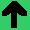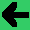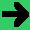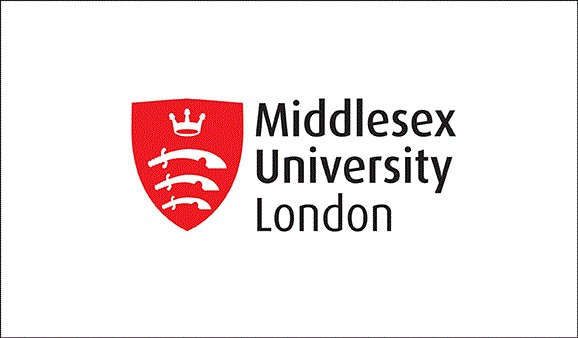# Lab 20: Nearest Neighbour Clustering

• Grab the data set from here. which is the S1 of S-sets example.
• Write a program (C# works) to cluster these items.
1. Read the data into an array. (2 points)
2. Set K points randomly (you can try K=15 or 20). (2 points)
3. Assign each point to one of the K points. (1 point)
4. Move K to the centre of its points. (1 point)
5. Repeat until none change. (2 points)
6. Print result. (1 point)
7. Compare (manually using excel ok) result. (1 point)
• If you read the datasets file, you'll notice that there are 15 clusters.
• You'll want to start by reading in the data.
• I used kMeans with K = 15.
• On my first test, I got 3372/5000. (Some clusters weren't really used.)
• I used excel to measure (the answers are in datasets above).
• Can you measure?
• I did have problems with the distance and squares overflowing the fields. So, I had to decompose the distance equation into parts and combine them with floats. (That took longer than the rest of the problem.)